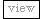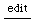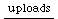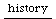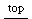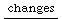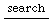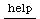## Understanding Assignment

=
Assignment is often a source of misconceptions, specially as this differs in different
languages and influence the design and structure of the whole language.
One of the sources of misunderstanding is that assignment is often symbolized in a similar way like equality.

in sc
= means assignment (by value)
== means equality, can be defined freely
=== means identity, this is found out by the hash ids of the objects

Another source of misunderstanding is the double meaning of a variable name in programming syntax:

z = z + 1;

the left z means a place where a value can be stored and the right z means the value that is stored in z.

Here is a couple of examples in sc that show demonstrate this: passByValue.html
(jrh)

"Most descriptions of imperative programming languages are tied to
hardware and implementation considerations where a name is bound
to an address, a variable to a storage cell, and a value to a bit pattern.

Thus, a name is tied to two bindings, a binding to a location and to a value.
The location is called the l-value and the value is called the r-value.
The necessity for this distinction follows from the implementation of the assignment.

For example,

X := X+2

the X on the left of the assignment denotes a location while the X on the right hand side
denotes the value. Assignment changes the value at a location.

A variable may bebound to a hardware location at various times (see Discrete vs. Continuous Evaluation).
It may be bound at compile time (rarely), at load time (for languages with static allocation)
or at run time (for languages with dynamic allocation).
From the implementation point of view, variable declarations are used to determine
the amount of storage required by the program."
(from: Anthony A. Aaby , http://cs.wwc.edu/~aabyan/221_2/PLBOOK/Imperative.html)